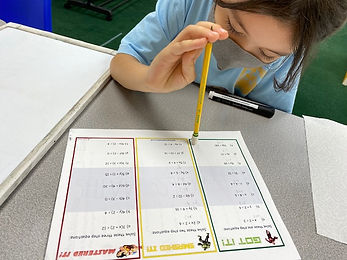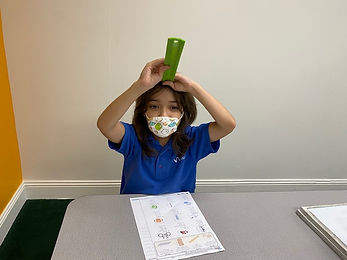## Ms. Dawn

### Target 1​

###### Lesson Type:

Continuation

Geometry

:

Coordinates

Use coordinates to find a location on a grid.

###### 1:

Understand that the first number in a coordinate pair indicates placement on the x-axis and the second indicates placement on the y-axis.

###### 2:

Identify the x- and y-axis on a coordinate plane.

###### 3:

Graph points in the first and second quadrant of the coordinate plane.

###### 4:

Become familiar with quadrant I of the coordinate plane and that all coordinate pairs will have positive numbers in this quadrant.

###### 5:

Become familiar with quadrant II of the coordinate plane and that the first number can be either positive or negative.

3rd

###### Vocabulary:

Coordinates, Coordinate plane, Quadrant, Pair, X-axis, Y-axis, Points, Plot, Ordered pair

Activities:

1. Students played a game called "Find Your Candy." Students placed a variety of candies on different plot points. Then, students try to guess where the candy is by calling out different ordered pairs.### Home Exploration

###### Guiding Questions:## Absent Students:

### Target 2

:

###### 1:

Identify the unknown information in problems.

3rd

###### Vocabulary:

Variable, Unknown, Represent, Equation

Activities:

1. Students were given a variety of equations with different operations and found the unknown number.

2. Students work their way up from bronze, silver, and gold problems. They use a variety of strategies to show their thinking.### Home Exploration

###### Guiding Questions:### Target 3

:

###### 1:

Understand how to measure length using a standard unit (centimeter, inches).

###### 2:

Understand units of measurement and which unit is most appropriate to measure which attribute.

###### 3:

Measure the length of objects by selecting appropriate measurement tools.

###### 4:

Estimate length based on understandings of unit measurements.

3rd

###### Vocabulary:

Measurement, Standard units, Centimeter, Inch, Foot, Yard, Mile, Millimeter, Meter, Kilometer, Estimate, Measurement tool

Activities:

1. Students were given a variety of pictures and measurements, They figured out which best measurement matches the pictures.### Home Exploration